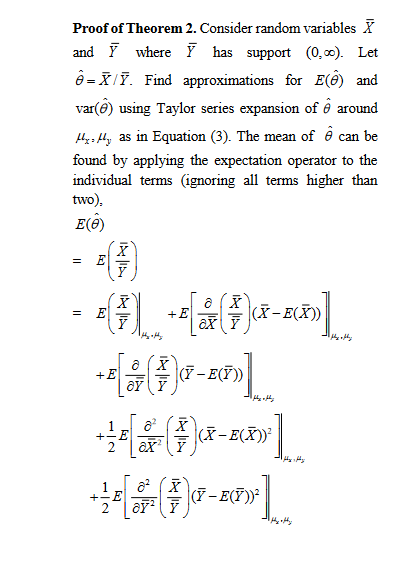# Confidence Interval for the Ratio of Bivariate Normal Means with a Known Coefficient of Variation

## Authors

• Wararit Panichkitkosolkul Department of Mathematics and Statistics, Faculty of Science and Technology, Thammasat University, Pathumthani 12121, Thailand.

## Keywords:

Interval estimation, Central tendency, Standardized measure of dispersion, Coverage probability, Expected length

## Abstract

An approximate confidence interval for the ratio of bivariate normal means with a known coefficient of variation is proposed in this paper. This application has the area of bioassay and bioequivalence when a scientist knows the coefficient of variation of a control group. The proposed confidence interval is based on the approximated expectation and variance of the estimator by the Taylor series expansion. A Monte Carlo simulation study was conducted to compare the performance of the proposed confidence interval with the existing confidence interval. The results showed that the simulation is considered confidence interval and the estimated coverage
probabilities close to the nominal confidence level for large sample sizes. The estimated coverage probabilities of the existing confidence interval are over estimated for all situations. In addition, the expected lengths of the proposed confidence interval are shorter than those of the existing confidence interval in all circumstances. When the sample size increases, the expected length become shorter. Therefore, our confidence interval presented in this paper performs well in terms of estimated coverage probability and the expected length in considering the simulation results. A comparison of the confidence intervals is also illustrated using an empirical application

## References

Bliss, C. I. (1935a). The calculation of the dose-mortality curve, Annals of Applied Biology,22(1), 134-167.

Bliss, C. I. (1935b).The comparison of dose-mortality data, Annals of Applied Biology, 22 (2),309-333.

Cox, C. P. (1985). Interval estimates for the ratio of the means of two normal populations with variances related to the means, Biometrics,

(1), 261-265.

Fieller, E. C. (1944). A fundamental formula in the statistics of biological assay and some applications, Quarterly Journal of Pharmacy

and Pharmacology, 17 (2), 117-123.

Fieller, E. C. (1954). Some problems in interval estimation, Journal of the Royal Statistical Society: Series B, 16 (2), 175-185.

Finney, D. J. (1947). The principles of the biological assay (with discussion), Journal of the Royal Statistical Society: Series B, 9 (1), 46-91.

Finney, D. J. (1965). The meaning of bioassay,Biometrics, 21 (4), 785-798.

Fisher, L. D. and Van Belle, G. (1993). A Methodology for the Health Sciences. New York: Wiley.

Ihaka, R. and Gentleman, R. (1996). R: A language for data analysis and graphics, Journal of Computational and Graphical Statistics, 5, 299-

Irwin, J. O. (1937). Statistical method applied to biological assay, Journal of the Royal Statistical Society: Series B, 4 (1), 1-60.

Kelly, G. E. 2000. The median lethal dose-design and estimation, Statistician, 50(1), 41-50.

Koschat, M. A. (1987). A characterization of the Fieller solution, Annals of Statistics, 15(1), 462-468.

Krishnamoorthy, K. and Xia, Y. (2007). Inferences on correlation coefficients: One-sample, independent and correlated cases, Journal of

Statistical Planning and Inferences, 137 (7), 2362-2379.

Lee, J. C. and Lin, S. H. (2004). Generalized confidence intervals for the ratio of means of two normal populations, Journal of Statistical

Planning and Inference, 123 (6), 49-60.

Lu, Y., Chow, S.C. and Zhu, S. (2014). In vivo and in vitro bioequivalence testing, Bioequivace & Bioavailability, 6 (2), 67-74.

Niwitpong, S., Koonprasert, S. and Niwitpong, S. (2011). Confidence intervals for the ratio of normal means with a known coefficient of

variation, Advances and Applications in Statistics, 25(1), 47-61.

Panichkitkosolkul, W. (2015, October 13-17). Approximate confidence interval for the ratio of normal means with a known coefficient of

variation, In V.N. Huynh, M. Inuiguchi, and T.Denoeux (Eds.), Integrated Uncertainty in Knowledge Modelling and Decision Making.

Paper presented at The 4th symposium on Integrated Uncertainty in Knowledge Modelling and Decision Making, Nha Trang, Vietnam

(pp.183-191). Switzerland: Springer

Srivastava, M. S. (1986). Multivariate bioassay, combination of bioassays and Fieller’s theorem, Biometrics, 42(1), 131-141.

Sun, W., Grosser, S. and Tsong, Y. (2016). Ratio of means vs. difference of means as measures of superiority, noninferiority, and averagebioequivalence, Journal of Biopharmaceutical Statistics,doi:10.1080/10543406.2016.1265536.

Vuorinen, J. and Tuominen, J. (1994). Fieller’s confidence intervals for the ratio of two means in the assessment of average bioequivalence from crossover data, Statistics in Medicine, 13 (23), 2531-2545.

Weerahandi, S. (1993). Generalized confidence intervals, Journal of the American Statistical Association, 88 (423), 899-905.2022-12-02

## How to Cite

Panichkitkosolkul, W. . (2022). Confidence Interval for the Ratio of Bivariate Normal Means with a Known Coefficient of Variation. Suan Sunandha Science and Technology Journal, 4(1), 1–13. Retrieved from https://li02.tci-thaijo.org/index.php/ssstj/article/view/293

## Section

Research Articles